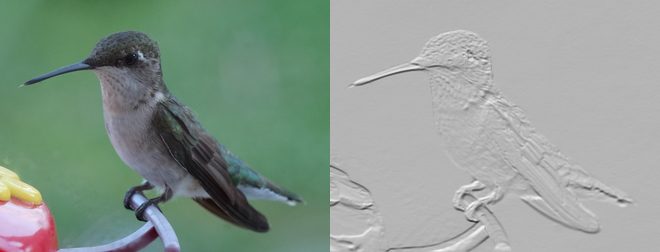# Transformation¶

Note

The image `transform.jpg` used in this docs is taken by Megan Trace, and licensed under CC BY-NC 2.0. It can be found the original photography from Flickr.

## Despeckle¶

New in version 0.5.0.

Despeckling is one of the many techniques you can use to reduce noise on a given image. Also see Enhance.

```from wand.image import Image

with Image(filename="hummingbird.jpg") as left:
with left.clone() as right:
right.despeckle()
left.extent(width=left.width*2)
left.composite(right, top=0, left=right.width)
left.save(filename="hummingbird-despeckle.jpg")
```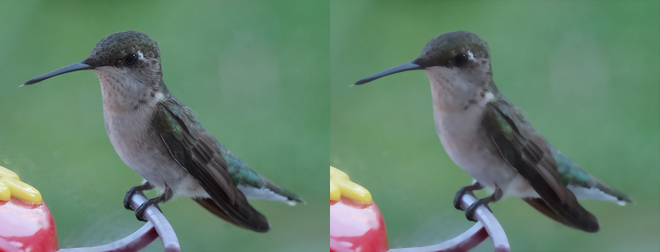## Edge¶

New in version 0.5.0.

Detects edges on black and white images with a simple convolution filter. If used with a color image, the transformation will be applied to each color-channel.

```from wand.image import Image

with Image(filename="hummingbird.jpg") as left:
with left.clone() as right:
right.transform_colorspace('gray')
right.edge(1)
left.extent(width=left.width*2)
left.composite(right, top=0, left=right.width)
left.save(filename="hummingbird-edge.jpg")
```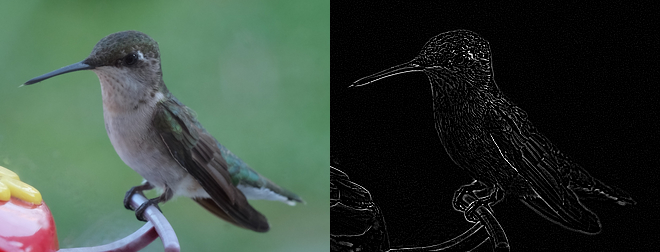## Emboss¶

New in version 0.5.0.

Generates a 3D effect that can be described as print reliefs. Like Edge, best results can be generated with grayscale image. Also see Shade.

```from wand.image import Image

with Image(filename="hummingbird.jpg") as left:
with left.clone() as right:
right.transform_colorspace('gray')
left.extent(width=left.width*2)
left.composite(right, top=0, left=right.width)
left.save(filename="hummingbird-emboss.jpg")
```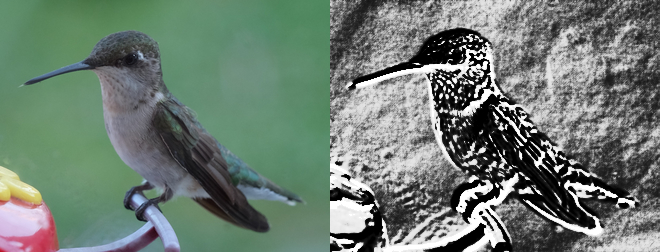## Enhance¶

New in version 0.5.0.

Reduce the noise of an image by applying an auto-filter. Also see Despeckle.

```from wand.image import Image

with Image(filename="hummingbird.jpg") as left:
with left.clone() as right:
right.enhance()
left.extent(width=left.width*2)
left.composite(right, top=0, left=right.width)
left.save(filename="hummingbird-enhance.jpg")
```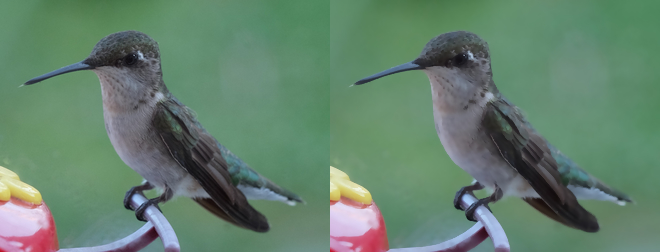## Flip and flop¶

New in version 0.3.0.

You can make a mirror image by reflecting the pixels around the central x- or y-axis. For example, where the given image `transform.jpg`:The following code flips the image using `Image.flip()` method:

```from wand.image import Image

with Image(filename='transform.jpg') as image:
with image.clone() as flipped:
flipped.flip()
flipped.save(filename='transform-flipped.jpg')
```

The image `transform-flipped.jpg` generated by the above code looks like:As like `flip()`, `flop()` does the same thing except it doesn’t make a vertical mirror image but horizontal:

```from wand.image import Image

with Image(filename='transform.jpg') as image:
with image.clone() as flopped:
flopped.flop()
flopped.save(filename='transform-flopped.jpg')
```

The image `transform-flopped.jpg` generated by the above code looks like:## Rotation¶

New in version 0.1.8.

`Image` object provides a simple method to rotate images: `rotate()`. It takes a `degree` which can be 0 to 359. (Actually you can pass 360, 361, or more but it will be the same to 0, 1, or more respectively.)

For example, where the given image `transform.jpg`:The below code makes the image rotated 90° to right:

```from wand.image import Image

with Image(filename='transform.jpg') as image:
with image.clone() as rotated:
rotated.rotate(90)
rotated.save(filename='transform-rotated-90.jpg')
```

The generated image `transform-rotated-90.jpg` looks like:If `degree` is not multiples of 90, the optional parameter `background` will help (its default is transparent):

```from wand.color import Color
from wand.image import Image

with Image(filename='transform.jpg') as image:
with image.clone() as rotated:
rotated.rotate(135, background=Color('rgb(229,221,112)'))
rotated.save(filename='transform-rotated-135.jpg')
```

The generated image `transform-rotated-135.jpg` looks like: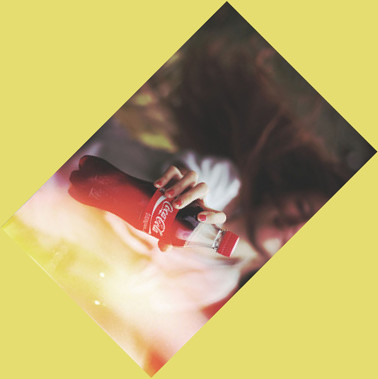New in version 0.5.0.

Creates a 3D effect by simulating light from source where `aziumth` controls the X/Y angle, and `elevation` controls the Z angle. You can also determine of the resulting image should be transformed to grayscale by passing `gray` boolean.

```from wand.image import Image

with Image(filename="hummingbird.jpg") as left:
with left.clone() as right: